# FedSQL Expressions

## Query Expressions and Subqueries

### Overview of Query Expressions

A query expression or query is one or more SELECT statements that produce a result set. Multiple SELECT statements can be combined by set operators.
Set operators (UNION, EXCEPT, and INTERSECT) combine columns from two queries based on their position in the referenced tables without regard to individual column names. Columns in the same relative position in the two queries must have the same data types. The column names of the tables in the first query become the column names of the result set.
A query expression with set operators is evaluated as follows.
• Each SELECT statement is evaluated to produce a virtual, intermediate result set.
• Each intermediate result set then becomes an operand that is linked with a set operator to form an expression (for example, A UNION B).
• If the query expression involves more than two SELECT statements, then the result from the first two becomes an operand for the next set operator and operand, such as (A UNION B) EXCEPT C, ((A UNION B) EXCEPT C) INTERSECT D, and so on.
• Evaluating a query expression produces a single output result set.
Note: There is no limit on the number of tables that you can reference in a FedSQL query. However, queries with a large number of table references can cause performance issues.

### Examples of Query Expressions

To understand how query expressions work, consider the following examples. The examples operate on two tables, named Numbers1 and Numbers2.
The following displays show the content of the tables.
Content of numbers1 Table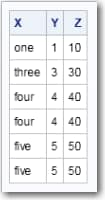Content of numbers2 Table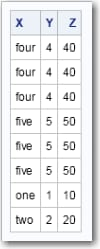The following example code specifies the EXCEPT operator:
```select * from numbers1 except select * from numbers2;
```
The EXCEPT operator returns values that exist in one table but not the other table in the comparison.
Output from EXCEPT Operation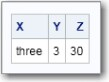The following example code specifies the INTERSECT operator:
`select * from numbers1 intersect select * from numbers2;`
The INTERSECT operator returns unique instances of values that the specified tables have in common.
Output from the INTERSECT Operation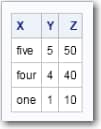The following example code specifies the UNION operator:
`select * from numbers1 union select * from numbers2;`
The UNION operator merges the unique values in the specified tables to display as one table.
Output from the UNION Operation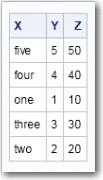### Overview of Subqueries

A subquery is a query expression that is nested as part of another query expression. It is specified within parenthesis and has the purpose of returning a value. A subquery can return atomic values (one column with one row in it – also known as a scalar query), row values (one row for one or many columns), or table values (one or many rows for one or many columns).
A subquery can be used in the SELECT, INSERT, UPDATE, and DELETE statements. The purpose of a subquery is to enable the contents of one table to influence a query or an action on another table.
Subqueries can appear in various places within a query:
• SELECT Statement
• WHERE Clause
• HAVING Clause
• FROM Clause
Scalar subqueries can be specified in all four locations, anywhere a scalar value can be used. Subqueries that return row values are typically specified in the WHERE clause. Subqueries that return table values are specified in the FROM clause.
A subquery can be dependent or independent of the outer query. When the information pursued in a subquery is dependent in some way on data known to the outer query, we say the data is correlated with the outer query. A correlated subquery typically references the data in the outer query with a correlation name or uses the EXISTS or IN predicate, and uses data from the outer query. The information retrieved by the correlated subquery will change if the data processed by the outer query changes. A correlated subquery is evaluated for each row identified by the outer query, making the subquery resource-intensive. Many correlated queries can be restated in terms of a join operation.
A subquery that is not dependent on the outer query is referred to as a non-correlated query. A non-correlated subquery does not interact much with the data being accumulated in the rest of the query. The non-correlated subquery is evaluated just once and the result used repeatedly in the evaluation of an outer query. Most importantly, the result of the subquery does not change if the data processed by the outer query changes.
Subqueries can be nested. If more than one subquery is used in a query expression, then the innermost query is evaluated first, then the next innermost query, and so on, moving outward.

### Examples of Correlated Subqueries

The following is an example of a correlated subquery that specifies the EXISTS predicate. It uses data from the example Employees and Depts tables. For a description of these tables, see Employees and
```select *
from employees e
where exists(select * from depts d
where d.deptno = e.dept
and e.pos <> 'Manager');
```
The EXISTS predicate stipulates to return information from the table in the outer query only for values that also exist in the inner query. Notice the second WHERE clause uses the column reference e.dept. If you look at the FROM clause in the outer query, you will see that e is a correlation name associated with the Employees table that is being used in the outer query.
Output of Correlated Query with EXISTS Predicate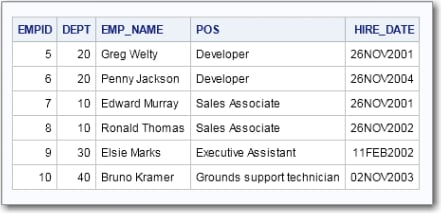Here is a more efficient way to write this query:
```select *
from employees
where dept in (select deptno from depts
where pos <> 'Manager');
```
This query does not require a correlation name. The contents of the Dept and Deptno columns are compared with the IN predicate. The query returns the same result as the previous query. Meanwhile, the inner query can be executed once and its results compared to each row in the Employees table.

### Examples of Scalar Subqueries

A scalar subquery returns one value for one column. As such, it is useful for aggregate queries.
Here is an example of a scalar subquery in the HAVING clause. The example uses the Employees and Depts tables that were used in Examples of Correlated Subqueries.
```select dept, count(emp_name)
from mybase.employees e
group by dept
having dept in (select deptno from mybase.depts);```
It is a simple request: count the number of employees in the Employees table, group the count by department, and return information only about departments having a matching value in the Deptno column of the Depts table.
Number of Employees by Department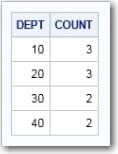The following is an example of a scalar subquery that is specified in the SELECT statement.
```select d.deptno,
d.deptname,
d.manager,
(select count (*) from employees e
where e.dept = d.deptno and
e.pos <> 'Manager') as Employees
from depts d;
```
The subquery counts the number of records in the Employees table, removes employee records that belong to managers, and then returns an aggregate value for each department code that has a match in both tables. That is, one value is returned for each department. It is also a correlated subquery.
Number of Employees by Manager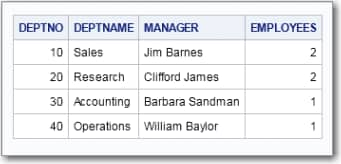Here is an example of a scalar subquery in the WHERE clause:
```select *
from depts d
where (select COUNT(*) FROM employees e
WHERE d.deptno = e.dept
and e.pos <> 'Manager') > 1;
```
As in the previous example, the subquery counts all of the rows in Employees table minus those that describe a manager and correlates them to the DeptNo column of the Depts table. However, the WHERE clause further qualifies the query to retrieve information only about departments that have more than one employee.
Departments with More Than One Employee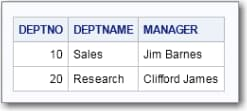The following is an example of a scalar subquery in the INSERT statement. This example uses data from the Densities example table, which has a column named Population. A new table, Summary, is created and populated with aggregated values from Densities table’s Population column. For more information about the Densities table, see Densities.
```create table summary (
sum_pop double having format comma12.,
max_pop double having format comma12.,
min_pop double having format comma12.,
avg_pop double having format comma12.
);

insert into summary (
sum_pop,
max_pop,
min_pop,
avg_pop)
values (
(select sum(population) from densities),
(select max(population) from densities),
(select min(population) from densities),
(select avg(population) from densities)
);
select * from summary;
```
Each subquery in the INSERT statement is a scalar subquery. Each subquery returns one value (the sum, maximum, minimum, and average values) from the data in one column, Population, to populate the new table.
Content of the Summary Table### Examples of Non-Correlated Queries

A non-correlated subquery is executed before the outer query and the subquery is executed only once. The data from the outer query and the subquery are independent and one execution of the subquery will work for all the rows from the outer query.
The examples in this section use data from the following three tables.
Table All1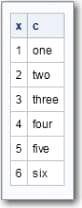Table One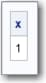Table Two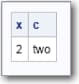This example code specifies a subquery in the SELECT statement. The subquery specifies to display only values from table All1 that exist in table One. Table All1 is not modified. The query affects outputted rows only:
`select * from all1 where x in (select x from one);`
Output of the SELECT Subquery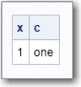The following example code specifies a subquery in the INSERT statement:
```insert into two select * from all1 where exists (select * from one);
select * from two;```
In this example, the content of table Two is expanded to include the contents of table All1 that also exists in table One.
Results of the INSERT Subquery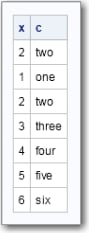This example code specifies a subquery in the DELETE statement:
```delete from all1 where x in (select x from one);
select * from all1;```
The code specifies to delete values from table All1 that match values in column X of table One.
Results of the DELETE Subquery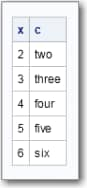The following example code specifies a subquery in an UPDATE statement:
```update all1 set x = (select x from two where c = 'one');
select * from all1;```
Column X in table All1 is updated with the value of column X in table One.
Results of the UPDATE Subquery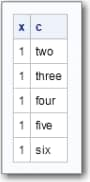A non-correlated subquery allows you select, insert, delete, and modify unrelated blocks of data between two or more tables.

## FedSQL Value Expressions

### Numeric Value Expressions

Numeric value expressions enable you to compute numeric values by using addition (+), subtraction (–), multiplication (*), and division (/) operators. Numeric values can be numeric literals. These values can also be column names, variables, or subqueries as long as the column names, variables, or subqueries evaluate to a numeric value.
The data type of the result of a numeric value expression is based on the data type of the operands.
Here are examples of numeric value expressions.
• –6
• salary * 1.07
• cost + (exp – discount)

### Row Value Expressions

A row value expression, or row value constructor, is one or more value expressions enclosed in parentheses. Multiple value expressions are separated by commas.
A row value constructor can contain the following values.
• value-expression
• NULL
• DEFAULT
• row-subquery
• (row-value-constructor, row-value-constructor, …)
• ROW (row-value-constructor, row-value-constructor, …)
NULL makes the value for the corresponding column in the table null. DEFAULT makes the value for the corresponding column the default value. ARRAY[ ] is valid only if the destination is an array and creates an empty array. The row constructor values other than NULL, DEFAULT, and ARRAY[ ] can be simple values or value expressions.
A row value constructor operates on a list of values or columns rather than a single value or column. You can operate on an entire row at a time or a subset of a row.
Here is an example where you can use row value constructors in the INSERT statement to add multiple values to a table.
```INSERT INTO inventory
(prodname, qty, price)
VALUES ('rice', 3849, .37);```
This example uses row value constructors in the WHERE clause to compare column values.
```SELECT * FROM inventory
WHERE (inventory.prodname, inventory.price)
=
(competitor.prodname, inventory.price);```